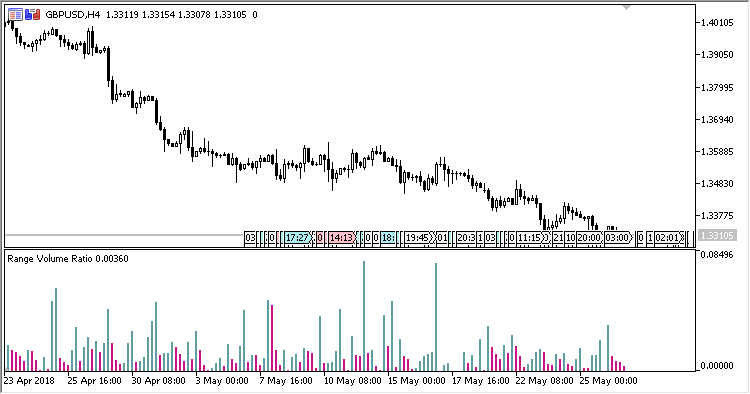# Range_Volume_Ratio – indicator for MetaTrader 5

The indicator shows in a separate window, as a histogram, the candlestick size / tick volume ratios:

1. Difference between Open and Close;
2. Difference between High and Low.

The indicator has one input parameter:

• Calculation type – type of calculations:
• Open/Close – difference between Open and Close;
• High/Low – difference between High and Low.

Calculations:

If the calculation type is Open/Close:

`RVR[i] = Abs(Open[i] - Close[i]) / Volume[i]`

If the calculation type is High/Low:

`RVR[i] = (High[i] - Low[i]) / Volume[i]`

where:

`Volume - the tick volume of the candlestick`Related Posts

Fig.1. Calculations by Open/Close

Fig.1. Calculations by High/Low